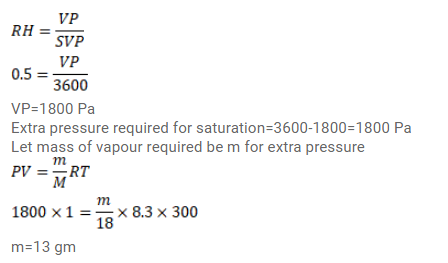# Use R=8.3 J/mol-K wherever required. The temperature and humidity ofQuestion:

Use $\mathrm{R}=8.3 \mathrm{~J} / \mathrm{mol}-\mathrm{K}$ wherever required.

The temperature and humidity of air are $27^{\circ} \mathrm{C}$ and $50 \%$ on a particular day. Calculate the amount of vapour that should be added to 1 cubic meter of air to saturate it. The saturation vapour pressure at $27^{\circ} \mathrm{C}=3600$ $\mathrm{Pa}$.

Solution: